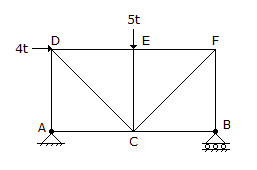# Civil Engineering - Theory of Structures - Discussion

### Discussion :: Theory of Structures - Section 1 (Q.No.17)

17.

The force in BF of the truss shown in given figure, is[A]. 4t tension [B]. 4t compression [C]. 4.5t tension [D]. 4.5t compression [E]. zero.

Answer: Option D

Explanation:

No answer description available for this question.

 Patel said: (Aug 17, 2013) Reactions are va+ab = 5t. Moment at A= 4t*x+5t*x-vb*2x = 0. So 9t = 2vb. vb = 4.5t. So force in compression member BF = 4.5t compression.

 P.K. Nayak said: (Jul 29, 2016) Taking moment about A. Vb * 2 = 5 * 1 + 4 * 1. => Vb = 4.5T Upward. Considering joint B Fbc = 0 & Fbf = 4.5T c.

 Krunal said: (Apr 15, 2017) How to determine it as compression or tension?

 Manoj Paridwal said: (Sep 11, 2017) Is sign is - then compression? If sign positive then tension.

 Abhi said: (Aug 21, 2018) How to consider the length of this? Please explain me in detail.

 Nath said: (Oct 2, 2018) Here, Assume x as the length of each segment.

 Gajanan Todeti said: (Sep 19, 2019) Answer is A) 4T tension.

 Michael said: (Apr 20, 2021) Why zero can't be? Explain, please.

#### Post your comments here:

Name *:

Email   : (optional)

» Your comments will be displayed only after manual approval.

#### Current Affairs 2021

Interview Questions and Answers